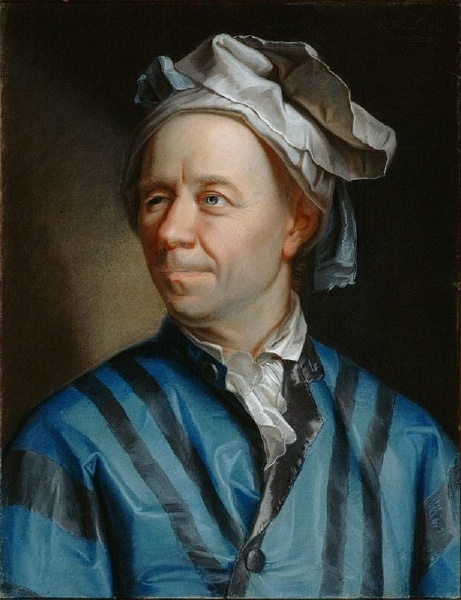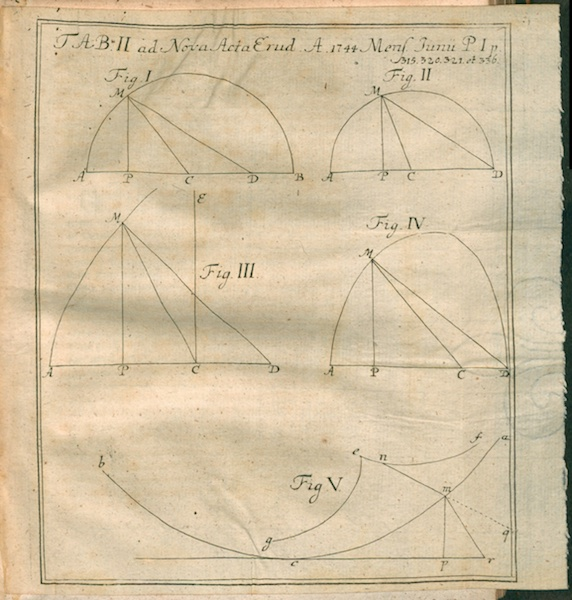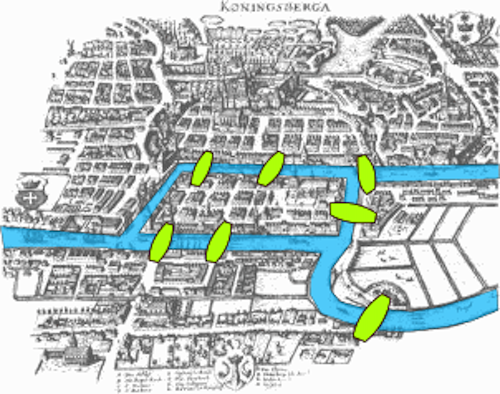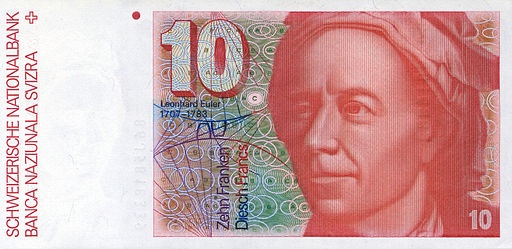# Happy Birthday, Leonhard Euler

April 15, 2018

Prolific and profound, Leonhard Euler is considered one of the greatest mathematical scientists of all time. He made formative contributions to whole branches of mathematics, among them infinitesimal calculus, graph theory, and topology. Best known for his eponymous formula and identity equation, part of his genius was the ability to apply equations to the world around him and explain scientific concepts in terms that a layperson could understand.

### Leonhard Euler, Promising Prodigy

Leonhard Euler (pronounced “oiler”) was born on April 15, 1707, in Basel, Switzerland. By age 13, he was already studying at the University of Basel, and by 16, he earned a Master of Philosophy degree. During his time at the university, he studied intensively with family friend and famed mathematician Johann Bernoulli, who encouraged Euler to pursue a career in mathematics.

Euler wrote a dissertation a few years later on the propagation of sound, titled De Sono, which was the first of many published mathematical works. In fact, by the end of his lifetime, Euler’s total collected works filled 60 to 80 quarto volumes, a larger collection than anyone else’s in the field. More success soon followed: In 1727, Euler placed second in an annual Paris Academy prize problem competition, a prize he came to win a total of 12 times.A portrait of Leonhard Euler. Image in the public domain in the United States, via Wikimedia Commons.

After spending some time in St. Petersburg, Russia, as both a medic in the Russian Navy and a professor of physics at the Imperial Russian Academy of Sciences, Euler moved to Berlin, Germany, to accept a job offer from Frederick the Great of Prussia. Euler spent 25 years at the Berlin Academy before returning to Russia, and it was in Berlin where he wrote his most groundbreaking work.

### Adding Up Euler’s Mathematical Contributions

It’s hard to solve formulas or talk about mathematical theories without referencing Euler’s work in one way or another. Euler contributed to topics such as functions in number theory and angles that define rotations in space. It’s no wonder that Pierre-Simon Laplace reportedly said “Read Euler; he is the master of us all.”

There are two well-known numbers named after Euler:

1. The “e” mathematical constant in calculus stands for “Euler”, and is approximately equal to 2.71828
2. “Euler’s constant”, or γ (gamma), is approximately equal to 0.57721

While Euler published many textbooks, two he published in Berlin made him famous in his field: Introductio in analysin infinitorum (1748) on functions and Institutiones calculi differentialis (1755) on differential calculus.

In his textbooks, Euler was the first mathematician to introduce the concept of a function in mathematical analysis, as well as the first mathematician to write the function f as applied to the variable x: f(x).

Other notations created or popularized by Euler include:

• Sum or total of a set of numbers, denoted by the Greek letter Σ
• “Imaginary” unit, equal to the square root of -1, denoted by the letter i
• Ratio of a circle’s circumference to its diameter, denoted by the Greek letter π (popularized after originating from Welsh mathematician William Jones)
• Trigonometric functions for sine, cosine, tangent, cotangent, secant, and cosecant
• Constants; a, b, c
• Variables or unknowns in an equation; x, y, z

His attempts to standardize many of these symbols helped mathematicians collaborate and solve problems together. Euler himself collaborated quite often with famous mathematicians, including Joseph-Louis Lagrange.One of Euler’s illustrations from his famous paper on topology and graph theory, “The Solution of a Problem Relating to the Geometry of Position”, first published in 1744 in the scientific journal Acta Eruditorum. Image in the public domain in the United States, via Wikimedia Commons.

#### The Most Beautiful Equation

Euler is also known for developing Euler’s identity, the equality e+1 = 0. It shows a connection between the most fundamental numbers in math and is a case of Euler’s formula, eix = cos x + i sin x, which establishes the relationship between trigonometric functions and complex exponential functions.

What’s interesting about Euler’s identity is that it is considered the epitome of mathematical beauty. It was voted by Mathematical Intelligencer readers in 1988 as the “most beautiful” theorem and even won beauty contests in brain scans.

### Applied Mathematics and Letters to a German Princess

Euler solved many real-world problems analytically and developed efficient tools to address them. A famous example is a thought problem known as the Seven Bridges of Königsberg. Königsberg, Prussia (now Kaliningrad, Russia), is situated between both sides of the Pregolya river. The city includes two islands that, during Euler’s time, were connected to each other by seven bridges. The problem’s objective was to come up with a path through the city that only crosses each bridge once.

Euler was able to prove, via satisfactory analysis involving many tests and graphs, that the problem has no solution. His methods laid the foundations for graph theory and topology.The seven bridges of Königsberg, Prussia, shown in green. Image by Bogdan Giuşcă, based on a map of Königsberg in the public domain. Licensed under CC-BY-SA-3.0, via Wikimedia Commons.

Besides textbooks, Euler published a work that propelled him to stardom beyond the praise of his fellow mathematicians. In the early 1760s, Frederick the Great asked Euler to tutor his niece, Friederike Charlotte of Brandenburg-Schwedt, the Princess of Anhalt-Dessau. Euler wrote the princess over 200 letters, which were later compiled and published. In the letters, Euler discussed math and science in simplified terms, which made them wildly popular among the public because they helped make mathematical concepts easier to understand.

Later in life, Euler’s eyesight deteriorated, but it did not limit his prolific output. In fact, he said that his blindness made him less distracted so that he could focus on his work. In 1775, several years after losing his eyesight, Euler wrote an average of one mathematical paper per week!

### The Lasting Significance of Leonhard Euler

Euler opened up a world of possibility with his fundamental symbols and equations. He established many areas of mathematics and contributed to structural mechanics and astronomy, including the creation of accurate longitude tables. He also applied mathematics to optics and music theory.Euler on an old Swiss 10-franc banknote. Image in the public domain, via Wikimedia Commons.

For his many gifts to mathematics, Euler has been commemorated on a Swiss 10-franc banknote and on Swiss, German, and Russian postage stamps. For his contributions to astronomy, the asteroid 2002 Euler was named after him.

Today, let’s wish Leonhard Euler a happy birthday!